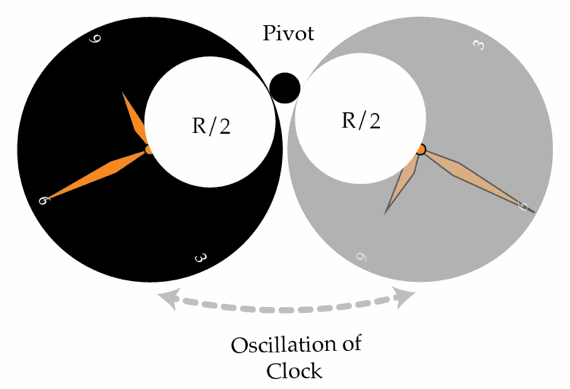# Damaged clock

A clock is made out of a disk of radius $R = 10~\mbox{cm}$ which is hung by a point on its edge and oscillates. All of a sudden, a circular part right next to the hanging point of radius $\frac{R}{2}$ falls off, but the clock continues oscillating. What is the absolute value of the difference in s between the periods of oscillation before and after the part fell off?Details and assumptions

• Gravitational acceleration is $g = 9.81~\mbox{m/s}^2$
• Amplitude of the vertical oscillations is small
• The axis of rotation of the disc is horizontal all the time
• The disc is homogeneous
×